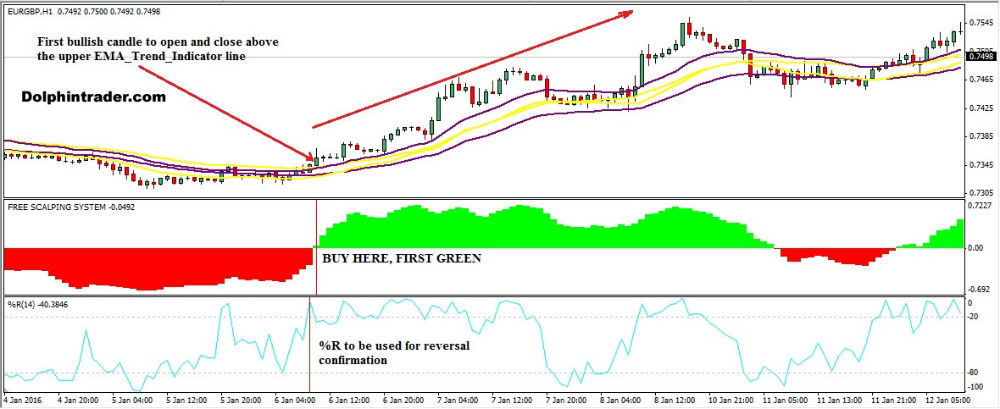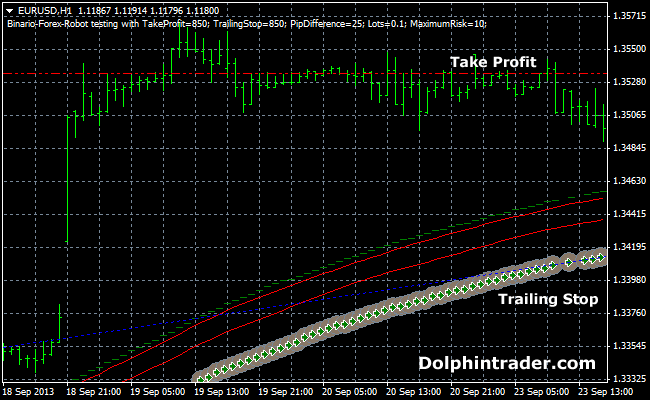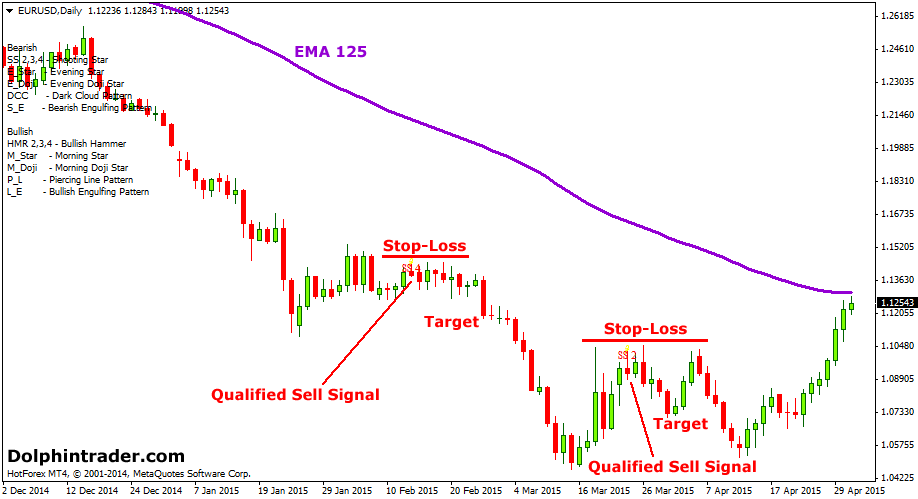#### Tag Archive

Tag Archives for " EMA "

## EMA Trend Indicator Simple Forex Day Trading StrategyThe EMA Trend Indicator Simple Day Trading Strategy is a forex trading strategy that is deployed for day trading. The custom indicator is designed after the Exponential Moving Averages using a cluster of four moving averages, where two of the lines represent the “Fast MA Period;” while the other two lines represents “Slow MA Period.” […]

## Double 144 Period EMA Forex RobotThis trading robot uses two 144 period EMA’s to initiate buy and sell trades. A trailing stop is used to lock in profits or reduce the trading risk for the open market position. As always, feel free to experiment with the EA input values until you find the optimal settings (take profit, trailing stop,…) that […]

## Shooting Star Forex StrategyThe Shooting Star forex strategy is made up of one reversal candlestick pattern and one exponential moving average for trend direction. This is a great reversal strategy used to trade short-term rallies found in a bearish market. The shooting star pattern is made up of one candlestick with a small body and little or no […]

## 3 EMA’s Forex Price Indicator

This indicator shows 3 exponential moving averages (short, medium, long) in a separated window below the main Metatrader 4 window. You can easily build cross-over moving average systems with this indicator. All EMA values can be changed from the inputs tab. Trading Signals Buy: Green short EMA above blue medium EMA and red long EMA. […]

## 2 EMA – 7 EMA Guppy Multi Moving Average Metatrader 4 Indicator

This is the eleventh GMMA forex indicator in a series of 11 indicators entirely based on the Guppy multi moving average. The RainbowMMA_10 consists of the following moving averages: 7 period exponential moving average, 6 period exponential moving average, 5 period exponential moving average, 4 period exponential moving average, 3 period exponential moving average and […]

## 8 EMA – 13 EMA Guppy Multi Moving Average Metatrader 4 Indicator

This is the tenth GMMA forex indicator in a series of 11 indicators entirely based on the Guppy multi moving average. The RainbowMMA_10 consists of the following moving averages: 13 period exponential moving average, 12 period exponential moving average, 11 period exponential moving average, 10 period exponential moving average, 9 period exponential moving average and […]

## 14 EMA – 23 EMA Guppy Multi Moving Average Metatrader 4 Indicator

This is the ninth GMMA forex indicator in a series of 11 indicators entirely based on the Guppy multi moving average. The RainbowMMA_09 consists of the following moving averages: 23 period exponential moving average, 21 period exponential moving average, 19 period exponential moving average, 17 period exponential moving average, 15 period exponential moving average and […]

## 25 EMA – 35 EMA Guppy Multi Moving Average Metatrader 4 Indicator

This is the eighth GMMA forex indicator in a series of 11 indicators entirely based on the Guppy multi moving average. The RainbowMMA_08 consists of the following moving averages: 35 period exponential moving average, 33 period exponential moving average, 31 period exponential moving average, 29 period exponential moving average, 27 period exponential moving average and […]